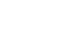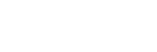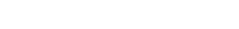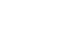# Chemistry Paper 1 Questions and Answers - KCSE Prediction Papers 2022

#### Instructions to Candidates.

• All working must be clearly shown.
• Mathematical tables and electronic calculators may be used.## Questions

1. Air is a mixture of several different gases, which parts of air;(3Mks)
1. Supports combustion?.......................................
2. Puts of a burning splint?...................................
3. Makes up almost 80% of air?.................................
2. In an experiment a test tube full of chlorine water was inverted in chlorine water to as shown in the diagram below and the set up was left in sunlight.After one day, a gas was found to have collected in the test tube.
1. Identify the gas(1Mk)
2. What will happen to the PH of the solution in the beaker after one day? Give an explanation. (2Mks
3. Draw the structure and give names of three alkanes having themolecular formula of C5H10. (3Mks)
4. Using electrons in the outermost energy level draw the dot(.)and cross(X )diagram for the molecules H2O and CH4(H=1, C=12 ) (2Mks)
5. The following chromatogram was obtained in an experiment to investigate the components present in certain dyes.1. Which two dyes when mixed would produce dyeA .(1Mk)
2. Identify the pure dye.(1Mk)
3. Define solvent front?(1Mk)
4. Indicate the solvent front in the diagram using the letter E.
6. A given element F has atomic number 14 and consist of isotopes as shown below.
 Isotopes G H J Isotopes Mass 28 29 30 Percentage abundance 92.2 4.7 3.4
1. Determine the relative atomic mass of element F. (2Mks)
2. State the group and the period to which element F belongs. (1Mk)
7. Hdrogen gas can be prepared by passing steam over heated magnesium ribbon as shown .
1. Write an equation for the reaction that produce hydrogen gas. (1Mk)
2. Explain why the delivery tube must be removed from beneath the water before heating is stopped.(1Mk)
3. Name the method of gas collection used in the experiment. Give a reason.(1Mk)
8.
1. State Charles’s law .(1Mk)
2. A gas occupies 450cm3 at 27°C.What volume would the gas occupy at 177°C.If its pressure remains constant? (Give the answer in Kelvin) (2Mks)
9. A certain match stick head contains potassium chlorate and sulphur. On striking, the two substances react to produce potassium chloride and sulphur(IV )oxide respectively.
1. Write an equation to show formation of sulphur (iv )oxide.(1Mks)
2. Explain the environmental effect of using such matches in large numbers.(2MKS)
10. The figure below shows a flame obtained from a Bunsen burner.1. Name the type of flame. (1Mk)
2. A match stick head was placed at region L will not ignite. Explain(1Mk)
3. Name region K .(1Mk)
11. Solutions can be classified as Acids, Bases or Neural. The table below shows solutions and their PH values.
 Solution PH values N   Q   P 1.5    7.0    13.0
1. Select any pairs that would react to form a solution of PH 7.0 (1Mk)
2. Identify twosolution that would react with Aluminium hydroxide. Explain. (2Mks)
12. The diagram below shows part of a synthetic polymer. Study it and answer the questions that follows;Draw the structure of the monomer (1Mk)
13. The diagram below shows the radiation emitted by a radioactive isotope.Name the radiations R, S and T.(3mks)
14.
1. Distinguish between a deliquescent and a hygroscopic substance.(2Mks)
2.
1. Give one use of a deliquescent substance In the laboratory. (1Mk)
2. Give one example of a hygroscopic substance
15. Study the flow diagram below and answer the question that follow.1. Write an equation for the reaction taking place in step 1. (1Mk)
2. Name solution V.(1Mk)
16.
1. state and exaplain two observations made when magnesium ribbon is lowered into a as jar full of carbon (iv) oxide (3mk)
2. Write a balanced chemical equation or the reaction that took place in a) above(1mk)
17. The energy level diagram below shows the effect of a catalyst on the reaction path.With reference to the energy level diagram, explain how a catalyst increases the rate of reaction. (2Mks)
18. The table shoes behaviour of metals R, X , Y and Z study it and answer the questions.
 Metal Appearance on exposure to air Reaction in water Reaction with dilute hydrochloric acid R   X   Y   Z Slowly tarnishes Slowly turns white No change No change Slow Vigorous Does not react No reaction Vigorous  Violet  Does not change  Reacts moderately
1. Arrange the metals in the order of reactivity starting with the most reactive (2Mks)
2. Name a metal which is likely to be ; (2Mks)
1. X:.............................
2. Y:.............................
19. The following chart below shows some properties of two allotropes of element P1. Name allotrope A(1Mk)
2. Write an equation to show formation of product P (1Mk)
3. What does 96°C represent? (1Mk)
20. Complete the following table by filling in the missing test and observations. (3Mks)
 No Gas Test Observation 1 Ammonia Put a moist red, then blue litmus into the gas 2 Sulphuric (V) oxide Paper turns green 3 Butene Add a drop of bromine water
21. An organic compound contains 24.24%carbon,4.04% hydrogen and the rest chlorine.If its relative molecular mass is 99.What is its molecular formula ?(C=12,H=1 ,Cl=35.5) (3Mks)
22. Study the diagram below and answer the questions that follows1. Define the term electrolysis.(2mks)
2. On the diagram, label the Anode and the Cathode. (1Mks)
3. Write the equation for reaction taking place at the Cathode. (1Mk)
23. Hardness of water may be removed by either boiling or addition of chemicals
1. Name the two types of water hardness. (2Mks)
2. A sample of river water was divided into three portions, the table shows the test carried out on the portion and observations made. Complete the table by filling the inferences. (3Mks)
 Test Observation Inference To the first portion,1cm3 of soap solution was added. No lather formed The second portion was boiled ,cooled and 1cm3 of soap solution was added. No lather formed To the third portion ,3cm3 of aqueous sodium carbonate was added ,the mixture filtered and 1cm3 of soap solution added to the filtrate. Lather formed immediately
24. The figure below shows an energy cycle diagram.1. Give the name of the enthalpy change H1 (1Mk)
2. Determine the value of H3 (2Mks)
25. Dry ammonia and dry oxygen were reacted as shown in the diagram.1. What is the purpose of the glass wool? (1Mk)
2. What product would be formed if red- hot platinum was introduced in to a mixture of ammonia and oxygen? (1Mk)
26. Study the diagram below.Identify apparatus A ,B and C. (3mks)
27. Explain why high temperature is required for Nitrogen to react with Oxygen. (2mks)## Marking Scheme

1. Air is a mixture of several different gases in air, which parts of air; (3mks)
1. Supports combustion. Oxygen
2. Puts off a burning splint?Carbon(VI)Oxide
3. Makes up almost 80% of air?Nitrogen
2. In an experiment a test tube full of chlorine water was inverted in chlorine water to as shown in the diagram below and the set up was left in sunlight.
After one day, a gas was found to have collected in the test tube.
1. Identify the gas........Oxygen...............................................................................................(1mk)
2. What will happen to the PH of the solution in the beaker after one day? Give an explanation. (2mks)
• PH decreases.
• Chlorine water /Hypochlorous acid/Chloric (V) acid decomposes to Hydrochloric acid which has a PH value less than 7.
3. Draw the structure and give names of three alkanes having the molecular formula of C5H10. (3mks)
4.
1. Using electrons in the outermost energy level draw the dot(.)and cross(X )diagram for the molecules H2O and CH4(H=1, C=12 ) (2mks)
1. H2O2. CH45. The following chromatogram was obtained in an experiment to investigate the components present in certain dyes.
1. Which two dyes when mixed would produce dyeA .(1mk)
• C and D
2. Identify the pure dye.
• B
3. Define solvent front? (1mk)
• The furthest the Solvent reaches on the adsorbent material.
4. Indicate the solvent front in the diagram using the letter E.
6. A diagram element F has atomic number 14 and consist of isotopes as shown below.
 Isotopes G H J Isotopes Mass 28 29 30 Percentage abundance 92.2 4.7 3.4
1. Determine the relative atomic mass of element F. (2mks)
2. State the group and the period to which element F belongs. (1mk)
• Group 4 and period 3 ½ m each
7. Hydrogen gas can be prepared by passing steam over heated magnesium ribbon as shown.
1. Write an equation for the reaction that produces hydrogen gas. (1mk)
Mg(s) + H2O → MgO(s) + H2(g)
2. Explain why the delivery tube must be removed from beneath the water before heating is stopped. (1mk)
• To avoid sucking back of water which may cause breaking of the boiling tube.
3. Name the method of gas collection used in the experiment a bove. Give a reason.
• Over water method .Hydrogen gas is slightly soluble in water (1mk)
8.
1. State Charle’s law (1mk)
• The volume of a given mass of gas is directly proportional to its absolute temperature at constant pressure
2. A gas occupies 450cm3 at 27°C.What volume would the gas occupy at 177°C.If its pressure remains constant?(Give the answer in Kelvin) (2mks)
9. A certain match stick head contains potassium chlorate and sulphur. On striking, the two substances react to produce potassium chloride and sulphur(IV )oxide respectively.
1. Write an equation to show formation of sulphur (VI )oxide. (1mk)
2. Explain the environmental effect of using such matches in large numbers. (2mks)
• A large volume of Sulphur (IV) Oxide shall be emitted into the atmosphere .The gas dissolves in atmospheric moisture/water to form Sulphuric (VI) acid which falls down as acid rain .The acid is destructive to human health ,metallic and stone structures and plants.
10. The figure below shows a flame obtained from a Bunsen burner.
1. Name the type of flame. (1mk)
• Non –luminous flame
2. A match stick head was placed at region L will not ignite. Explain (1mk)
• Is not hot /Unburnt gas region/has pure unburnt laboratory gas
3. Name region K . (1mk)
• Grenish –blue region
11. Solutions can be classified as Acids, Bases or Neural. The table below shows solutions and their PH values.
1. Select any pairs that would react to form a solution of PH 7.0 (1mk)
• N and P
2. Identify two solutions that would react with Aluminium hydroxide. Explain. (2mks)
• Nand P
• Aluminium hydroxide displays amphoterism,thus reacts with acids and bases
12. The diagram below shows part of a synthetic polymer. Study it and answer the questions that follow;
1. Draw the structure of the monomer (1mk)
13. The diagram below shows the radiation emitted by a radioactive isotope.
R: .........Beta particles
S: ..........Gamma rays
T: ..........Alpha particles
14.
1. Distinguish between a deliquescent and a hygroscopic substance. (2mks)
• Deliquescent substance absorbs water from the atmosphere to form a solution while a hygroscopic substance absorbs water from the atmosphere but do not dissolve to form a solution .
2.
1. Give one use of a deliquescent substance In the laboratory. (1mk)
• Drying agent during preparation of gases
2. Give one example of a hygroscopic substance
• Potassium nitrate/anhydrous Cobalt (II) chloride/anhydrous Copper (II) sulphate
15. Study the flow diagram below and answer the question that follow.
1. Write an equation for the reaction taking place in step 1. (1mk)
4 Fe(s) + 3O2(g) → 2Fe2O3(s)
2. Name solution V. (1mk)
• Iron (II) Chloride
16.
1.
• white powder of magnesium oxide is formed
• black specks of carbon are seen
2. 2 Mg + CO2 → 2MgO + C
17. The energy level diagram below shows the effect of a catalyst on the reaction path.
1. What does point M represent? (1mk)
• Maximum energy required to initiate a reaction/activation energy
2. With reference to the energy level diagram, explain how a catalyst increases the rate of reaction. (2mks)
• A catalyst lowers the activation and increases the rate of collisions of the reacting particles.
18. The table shoes behaviour of metals R, X , Y and Z study it and answer the questions.
 Metal Appearance on exposure to air Reaction in water Reaction with dilute hydrochloric acid R   X   Y   Z Slowly tarnishes Slowly turns white No change No change Slow Vigorous Does not react No reaction Vigorous  Violet  Does not change  Reacts moderately

1.  Arrange the metals in the order of reactivity starting with the most reactive (2mks)
• R,X,Z,Y.
2. Name a metal which is likely to be ; (2mks)
1. X:Sodium metal
2. Y:Copper metal
19. The following chart below shows some properties of two allotropes of element P.
1. Name allotrope A (1mk)
• Rhombic Sulphur
2. Write an equation to show formation of product P (1mk)
3. What does 96°C represent?
• Transition temperature
20. Complete the following table by filling in the missing test and observations.(3Mks)
 No Gas Test Observation 1 Ammonia Put a moist red, then blue litmus into the gas Red litmus turns blue while blue remains blue 2 Sulphuric (V) oxide Potassium dichromate paper Paper turns green 3 Butene Add a drop of bromine water Yellow bromine water turns colourless
21. .An organic compound contains 24.24%carbon,4.04% hydrogen and the rest chlorine.If its relative molecular mass is 99.What is its molecular formula ?(C=12,H=1,Cl:35.5) (3mks)
22. Study the diagram below and answer the questions that follow
1. Define the term electrolysis. (1mk)
• Is the process of decomposing an electrolyte by passing an electric current through it
2. On the diagram, label the Anode and the Cathode. (2mks)
3. Write the equation for reaction taking place at the Cathode. (1mk)
23. Hardness of water may be removed by either boiling or addition of chemicals
1. Name the two types of water hardness. (2mks)
• Permanent water hardness
• Temporary water hardness
2. A sample of river water was divided into three portions, the table shows the test carried out on the portion and observations made. Complete the table by filling the inferences. (3mks)
 Test Observation Inference To the first portion,1cm3 of soap solution was added. No lather formed Hard water The second portion was boiled ,cooled and 1cm3 of soap solution was added. No lather formed Permanent hardness To the third portion ,3cm3 of aqueous sodium carbonate was added ,the mixture filtered and 1cm3 of soap solution added to the filtrate. Lather formed immediately Ca2+,Mg2+ ions are removed through precipitation
24. The figure below shows an energy cycle diagram.
1. Give the name of the enthalpy change H1
• Enthalpy of Combustion of hydrogen
2. Determine the value of H3 (2mks)
25. Dry ammonia and dry oxygen were reacted as shown in the diagram.
1. What is the purpose of the glass wool? (1mk)
• To spread out the Oxygen gas.
2. What product would be formed if red- hot platinum was introduced in to a mixture of ammonia and oxygen? (1mk)
• Forms NO, Nitrogen (II) Oxide and steam
26. Study the diagram below.
Identify apparatus A ,B and C. (3mks)
• A: Thistle funnel
• B: Delivery tube
27. Explain why Nitrogen gas requires a lot of heat when reacting with Oxygen. (2mks)
• Because of this high bond energy the activation energy for reaction of molecular nitrogen is usually very high, causing nitrogen to be relatively inert to most reagents under ordinary conditions.
(80 MARKS)

• ✔ To read offline at any time.
• ✔ To Print at your convenience
• ✔ Share Easily with Friends / Students